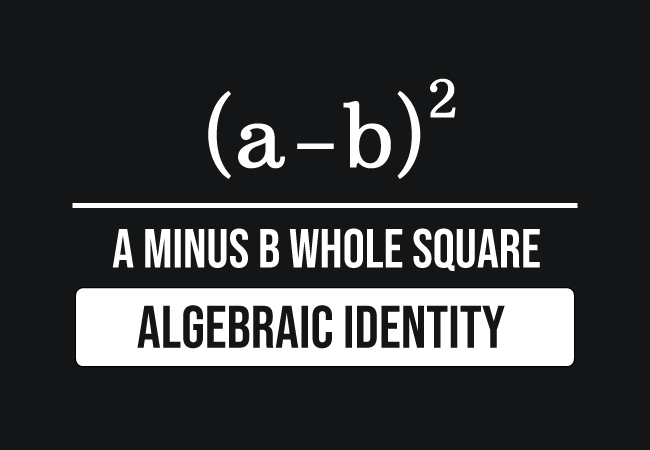# a minus b whole square identity

## Formula

$(a-b)^2 \,=\, a^2+b^2-2ab$

### Introduction

Let’s take two literals $a$ and $b$, and assume that they denote two quantities in algebraic form.

1. Firstly, subtract the literal $b$ from $a$ but it is not possible because the values of them are unknown. However, their difference is mathematically written as a binomial $a-b$.
2. Now, the square of the binomial $a$ minus $b$ is expressed as $(a-b)^2$ in mathematics.The expression $(a-b)^2$ is basically an algebraic expression, and we usually read it in the following two ways in mathematics.

1. The $a$ minus $b$ whole square.
2. The square of $a$ minus $b$.

Let’s learn what $a$ minus $b$ whole square is from its expansion.

#### Expansion

$(a-b)^2 \,=\, a^2-2ab+b^2$

$a$ minus $b$ whole square is expanded as $a$ square minus two times the product of $a$ and $b$ plus $b$ square.

#### Verification

Let’s take $a = 3$ and $b = 1$.

Now, find the value of expression $(a-b)^2$ by substituting the values of $a$ and $b$.

$\implies$ $(3-1)^2$ $\,=\,$ $(2)^2$ $\,=\,$ $4$

Similarly, evaluate the expression $a^2-2ab+b^2$ by substituting the values of $a$ and $b$.

$\implies$ $3^2-2(3)(1)+1^2$ $\,=\,$ $9-6+1$ $\,=\,$ $4$

Now, compare the values of both sides of the algebraic equation to verify their equality numerically.

$\,\,\,\therefore\,\,\,\,\,\,$ $(3-1)^2$ $\,=\,$ $4$ $\,=\,$ $3^2-2(3)(1)+1^2$

The square of $a-b$ is true for all the values of $a$ and $b$. It is defined in algebraic form. Hence, the $a$ minus $b$ whole square is generally called an algebraic identity and it can be used as a formula in mathematics.

#### Uses

The $a-b$ whole square identity is mainly used in two different cases in mathematics.

##### Expansion

$\implies$ $(a-b)^2 \,=\, a^2+b^2-2ab$

##### Simplification

$\implies$ $a^2+b^2-2ab \,=\, (a-b)^2$

#### Examples

Let us learn how to use the formula of $a$ minus $b$ whole square from the following simple examples.

$(1) \,\,\,$ Expand $(2x-5y)^2$

Take $a = 2x$ and $b = 5y$, and replace them in the expansion of the formula for calculating the value in mathematical form.

$\implies$ $(2x-5y)^2$ $\,=\,$ $(2x)^2+(5y)^2-2(2x)(5y)$

$\implies$ $(2x-5y)^2$ $\,=\,$ $4x^2+25y^2-2 \times 2x \times 5y$

$\,\,\, \therefore \,\,\,\,\,\,$ $(2x-5y)^2$ $\,=\,$ $4x^2+25y^2-20xy$

$(2) \,\,\,$ Simplify $9m^2+16n^2-24mn$

$\implies$ $9m^2+16n^2-24mn$ $\,=\,$ $(3m)^2+(4n)^2-24mn$

$\implies$ $9m^2+16n^2-24mn$ $\,=\,$ $(3m)^2+(4n)^2-2 \times 3 \times 4 \times m \times n$

$\implies$ $9m^2+16n^2-24mn$ $\,=\,$ $(3m)^2+(4n)^2-2 \times 3 \times m \times 4 \times n$

$\implies$ $9m^2+16n^2-24mn$ $\,=\,$ $(3m)^2+(4n)^2-2 \times (3m) \times (4n)$

$\implies$ $9m^2+16n^2-24mn$ $\,=\,$ $(3m)^2+(4n)^2-2(3m)(4n)$

Take $a = 3m$ and $b = 4n$. Now, simplify the algebraic expression by the $(a-b)^2$ algebraic identity

$\,\,\, \therefore \,\,\,\,\,\,$ $9m^2+16n^2-24mn$ $\,=\,$ $(3m-4n)^2$

#### Proofs

The formula of square of $a-b$ can be derived mathematically in two distinct methods.

##### Algebraic method

Learn how to derive the $a-b$ whole square identity by multiplying the binomials.

##### Geometric method

Learn how to prove the $a-b$ whole squared formula by the areas of geometric shapes.

###### Recommended
Latest Math Topics
Jun 26, 2023
Jun 23, 2023

Latest Math Problems
Jul 01, 2023
Jun 25, 2023
###### Math Questions

The math problems with solutions to learn how to solve a problem.

Learn solutions

Practice now

###### Math Videos

The math videos tutorials with visual graphics to learn every concept.

Watch now

###### Subscribe us

Get the latest math updates from the Math Doubts by subscribing us.